Know more about the Repeaters Batch Know more about the Repeaters Batch

# Chemical Bonding

## What is Chemical Bonding?

Chemical Bonding refers to the formation of a chemical bond between two or more atoms, molecules, or ions to give rise to a chemical compound. These chemical bonds are what keep the atoms together in the resulting compound.

### JEE Main 2021 LIVE Chemistry Paper Solutions 24-Feb Shift-1 Memory-Based### Table of Content

The attractive force which holds various constituents (atom, ions, etc.) together and stabilizes them by the overall loss of energy is known as chemical bonding. Therefore, it can be understood that chemical compounds are reliant on the strength of the chemical bonds between its constituents; The stronger the bonding between the constituents, the more stable the resulting compound would be.

The opposite also holds true; if the chemical bonding between the constituents is weak, the resulting compound would lack stability and would easily undergo another reaction to give a more stable chemical compound (containing stronger bonds). To find stability, the atoms try to lose their energy.

Whenever matter interacts with another form of matter, a force is exerted on one by the other. When the forces are attractive in nature, the energy decreases. When the forces are repulsive in nature, the energy increases. The attractive force that binds two atoms together is known as the chemical bond.

## Important Theories on Chemical Bonding

Albrecht Kössel and Gilbert Lewis were the first to explain the formation of chemical bonds successfully in the year 1916. They explained chemical bonding on the basis of the inertness of noble gases.

### Lewis Theory of Chemical Bonding

• An atom can be viewed as a positively charged ‘Kernel’ (the nucleus plus the inner electrons) and the outer shell.
• The outer shell can accommodate a maximum of eight electrons only.
• The eight electrons present in the outer shell occupy the corners of a cube which surround the ‘Kernel’.
• The atoms having octet configuration, i.e. 8 electrons in the outermost shell, thus symbolize a stable configuration.
• Atoms can achieve this stable configuration by forming chemical bonds with other atoms. This chemical bond can be formed either by gaining or losing an electron(s) (NaCl, MgCl2) or in some cases due to the sharing of an electron (F2).
• Only the electrons present in the outer shell, also known as the valence electrons take part in the formation of chemical bonds. Gilbert Lewis used specific notations better known as Lewis symbols to represent these valence electrons.
• Generally, the valency of an element is either equal to the number of dots in the corresponding Lewis symbol or 8 minus the number of dots (or valence electrons).

Lewis symbols for lithium (1 electron), oxygen (6 electrons), neon (8 electrons) are given below:Here, the number of dots that surround the respective symbol represents the number of valence electrons in that atom.

### Kossel’s theory of Chemical Bonding

• Noble gases separate the highly electronegative halogens and the highly electropositive alkali metals.
• Halogens can form negatively charged ions by gaining an electron. Whereas alkali metals can form positively charged ions by losing an electron.
• These negatively charged ions and positively charged ions have a noble gas configuration that is 8 electrons in the outermost shell. The general electronic configuration of noble gases (except helium) is given by ns2np6.
• As unlike charges attract each other these unlike charged particles are held together by a strong force of electrostatic attraction existing between them. For example, MgCl2, the magnesium ion, and chlorine ions are held together by force of electrostatic attraction. This kind of chemical bonding existing between two unlike charged particles is known as an electrovalent bond.

### Explanation of Kossel Lewis Approach

In 1916 Kossel and Lewis succeeded in giving a successful explanation based upon the concept of an electronic configuration of noble gases about why atoms combine to form molecules. Atoms of noble gases have little or no tendency to combine with each other or with atoms of other elements. This means that these atoms must be having stable electronic configurations.

Due to the stable configuration, the noble gas atoms neither have any tendency to gain or lose electrons and, therefore, their combining capacity or valency is zero. They are so inert that they even do not form diatomic molecules and exist as monoatomic gaseous atoms.

## Types of Chemical Bonds

When substances participate in chemical bonding and yield compounds, the stability of the resulting compound can be gauged by the type of chemical bonds it contains.

The type of chemical bonds formed vary in strength and properties. There are 4 primary types of chemical bonds which are formed by atoms or molecules to yield compounds. These types of chemical bonds include:

• Ionic Bonds
• Covalent Bonds
• Hydrogen Bonds
• Polar Bonds

These types of bonds in chemical bonding are formed from the loss, gain, or sharing of electrons between two atoms/molecules.

### Ionic Bonding

Ionic bonding is a type of chemical bonding which involves a transfer of electrons from one atom or molecule to another. Here, an atom loses an electron which is in turn gained by another atom. When such an electron transfer takes place, one of the atoms develops a negative charge and is now called the anion.

The other atom develops a positive charge and is called the cation. The ionic bond gains strength from the difference in charge between the two atoms, i.e. the greater the charge disparity between the cation and the anion, the stronger the ionic bond.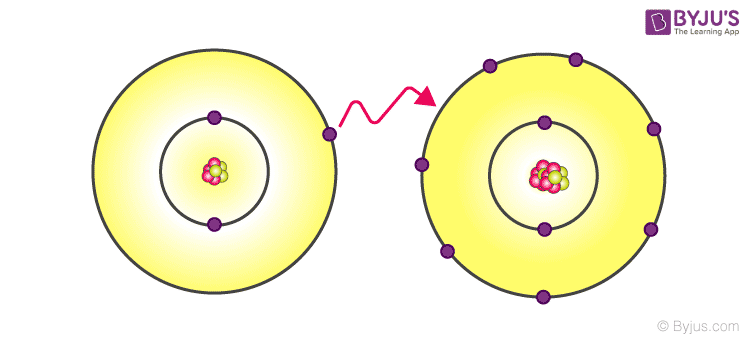Types of Chemical Bonds – Ionic bonding

### Covalent Bonding

covalent bond indicates the sharing of electrons between atoms. Compounds that contain carbon (also called organic compounds) commonly exhibit this type of chemical bonding. The pair of electrons which are shared by the two atoms now extend around the nuclei of atoms, leading to the creation of a molecule.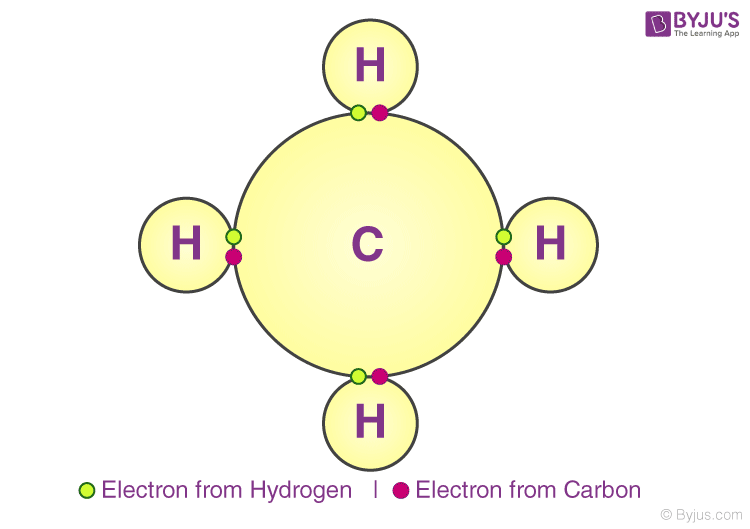Covalent Bonding

### Polar Covalent Bonding

Covalent bonds can be either be Polar or Non-Polar in nature. In Polar Covalent chemical bonding, electrons are shared unequally since the more electronegative atom pulls the electron pair closer to itself and away from the less electronegative atom. Water is an example of such a polar molecule.

A difference in charge arises in different areas of the atom due to the uneven spacing of the electrons between the atoms. One end of the molecule tends to be partially positively charged and the other end tends to be partially negatively charged.

### Hydrogen Bonding

Compared to ionic and covalent bonding, Hydrogen bonding is a weaker form of chemical bonding. It is a type of polar covalent bonding between oxygen and hydrogen wherein the hydrogen develops a partial positive charge. This implies that the electrons are pulled closer to the more electronegative oxygen atom.

This creates a tendency for the hydrogen to be attracted towards the negative charges of any neighbouring atom. This type of chemical bonding is called a hydrogen bond and is responsible for many of the properties exhibited by water.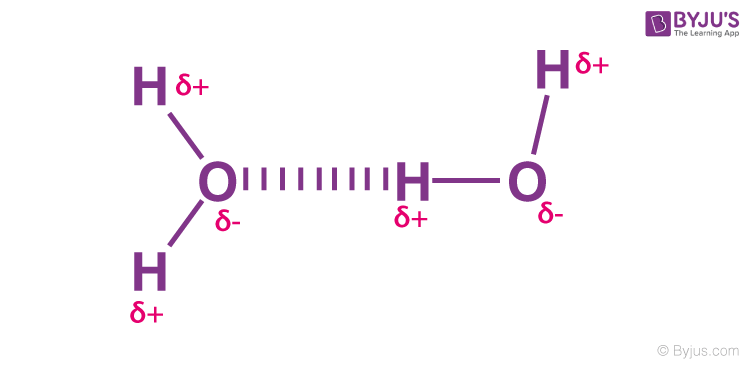Hydrogen Bonding

## What is Ionic Bond?

The bond formed as a result of strong electrostatic forces of attraction between a positively and negatively charged species is called an electrovalent or ionic bond. The positively and negatively charged ions are aggregated in an ordered arrangement called the crystal lattice which is stabilized by the energy called the Lattice enthalpy.

#### Conditions for the formation of an Ionic Bond

• The low ionization energy of the atom forming the cation.
• High electron gain enthalpy of the atom forming the anion.
• High negative lattice enthalpy of the crystal formed.

Generally, the ionic bond is formed between a metal cation and non-metal anion.

## Chemical Bonding and Molecular Structure Rapid Revision## Writing Lewis Structures

The following steps are adopted for writing the Lewis dot structures or Lewis structures:

Step 1: Calculate the number of electrons required for drawing the structure by adding the valence electrons of the combining atoms. For Example, in methane, CH4 molecule, there are 8 valence electrons (in which 4 belongs to carbon while other 4 to H atoms).

Step 2: Each negative charge i.e. for anions, we add an electron to the valence electrons and for each positive charge i.e. for cations we subtract one electron from the valence electrons.

Step 3: Using the chemical symbols of the combining atoms and constructing a skeletal structure of the compound, divide the total number of electrons as bonding shared pairs between the atoms in proportion to the total bonds.

Step 4: The central position in the molecule is occupied by the least electronegative atom. Hydrogen and fluorine generally occupy the terminal positions.

Step 5: After distributing the shared pairs of electrons for single bonds, the remaining electron pairs are used for multiple bonds or they constitute lone pairs.

The basic requirement is that each bonded atom gets an octet of electrons.

Example 1: Lewis formula for carbon monoxide, CO

Step 1: Counting the total number of valence electrons of carbon and oxygen atoms: C (2s22p2) + O (2s22p4) 4 + 6 = 10 that is, 4(C) + 6(O) = 10

Step 2: The skeletal structure of carbon monoxide is written as CO

Step 3: Drawing a single bond between C and O and completing octet on O, the remaining two electrons are lone pair on C.Step 4: This does not complete the octet of carbon, and hence we have a triple bond.Example 2: Lewis Structure of nitrite, NO2

Step 1: Counting the total number of valence electrons of one nitrogen atom, two oxygen atoms and the additional one negative charge (equal to one electron). Total Number of valence electrons is: N (2s22p3) + 2O (2s22p4) + 1 (negative charge) => 5+ 2(6) +1=18e

Step 2: The skeletal structure of nitrite ion is written as O-N-O

Step 3: Drawing a single bond between nitrogen and each oxygen atom: O – N – O

Step 4: Complete the octets of atoms.This structure does not complete octet on N if the remaining two electrons constitute of a lone pair on it. Therefore, we have a double bond between one N and one of the two O atoms. The Lewis structure isProblems:

1. Write the Lewis structure for the following.
2. CO32- b) CN c) SO52-

## Bond Characteristics

### Bond Length

During chemical bonding, when the atoms come closer to each other, the attraction takes place between them and the potential energy of the system keeps on decreasing till a particular distance at which the potential energy is minimum. If the atoms come more closer, repulsion starts and again the potential energy of the system begins to increase.

At equilibrium distance, the atoms keep on vibrating about their mean position. The equilibrium distance between the centres of the nuclei of the two bonded atoms is called its Bond length.

It is expressed in terms of an angstrom (A0) or picometer (pm). It is determined experimentally by x-ray diffraction or electron diffraction method or spectroscopic method. The bond length in chemical bonding is the sum of their ionic radii, in an ionic compound. In a covalent compound, it is the sum of their covalent radii. For a covalent molecule AB, the bond length is given by d= ra + rb

#### Factors Affecting the Bond length

• Size of the atoms: The bond length increases with increase in the size of the atom. HI > HBr > HCl > HF
• The multiplicity of Bond: The bond length decreases with an increase in bond order.
• Type of hybridization: A‘s’ orbital is smaller in size, greater the ‘s’ character, shorter is the bond length.

## Bond Enthalpy

When atoms come close together the energy is released due to the chemical bonding between them. The amount of energy required to break one mole of bonds of a type so as to separate the molecule into individual gaseous atoms is called bond dissociation enthalpy or Bond enthalpy. Bond enthalpy is usually expressed in KJ mol-1.

Greater is the bond dissociation enthalpy, greater is the bond strength. For diatomic molecules like H2, Cl2, O2, N2, HCl, HBr, HI the bond enthalpies are equal to their dissociation enthalpy.

In the case of polyatomic molecules, bond enthalpies are usually the average values, because the dissociation energy varies with each type of bond.

In H20, first O-H bond enthalpy = 502 KJ/mol; Second bond enthalpy = 427 KJ/mol Average bond enthalpy = (502 + 427) / 2 = 464.5 KJ/mol

### Factors Affecting Bond Enthalpy in Chemical Bonding

#### Size of the Atom

Greater the size of the atom, greater is the bond length and less is the bond dissociation enthalpy i.e. less is the bond strength during chemical bonding.

#### Multiplicity of Bonds

Greater is the multiplicity of the bond, greater is the bond dissociation enthalpy.

#### Number of Lone Pair of Electrons Present

More the number of lone pair of electrons present on the bonded atoms, greater is the repulsion between the atoms and thus less is the bond dissociation enthalpy of the chemical bond.

#### Bond Angle

A bond is formed by the overlap of atomic orbitals. The direction of overlap gives the direction of the bond. The angle between the lines representing the direction of the bond i.e. the orbitals containing the bonding electrons is called the bond angle.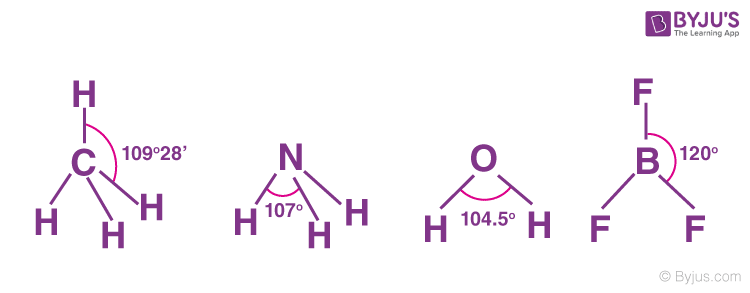Factors Affecting Bond Enthalpy in Chemical Bonding

#### Bond Order

In Lewis representation, the number of bonds present between two atoms is called the bond order. Greater the bond order, greater is the stability of the bond during chemical bonding i.e. greater is the bond enthalpy. Greater the bond order, shorter is the bond length.

## Resonance in Chemical Bonding

There are molecules and ions for which drawing a single Lewis structure is not possible. For example, we can write two structures of O3.In (A) the oxygen-oxygen bond on the left is a double bond and the oxygen-oxygen bond on the right is a single bond. In B the situation is just the opposite. The experiment shows, however, that the two bonds are identical.

Therefore neither structure A nor B can be correct. One of the bonding pairs in ozone is spread over the region of all three atoms rather than localized on a particular oxygen-oxygen bond. This delocalized bonding is a type of chemical bonding in which bonding pair of electrons are spread over a number of atoms rather than localized between two.Structures (A) and (B) are called resonating or canonical structures and (C) is the resonance hybrid. This phenomenon is called resonance, a situation in which more than one canonical structure can be written for a species. The chemical activity of an atom is determined by the number of electrons in its valence shell. With the help of the concept of chemical bonding, one can define the structure of a compound and is used in many industries for manufacturing products in which the true structure cannot be written at all.

Some other examples:

• CO32– ion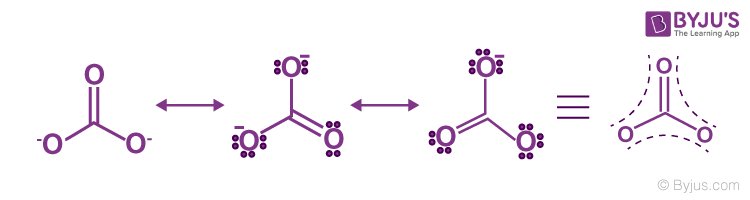• Carbon-oxygen bond lengths in carboxylate ion are equal due to resonance.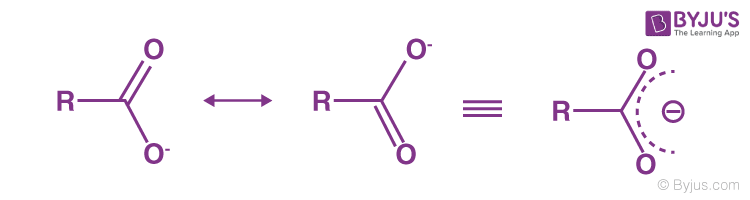• Benzene• Vinyl Chloride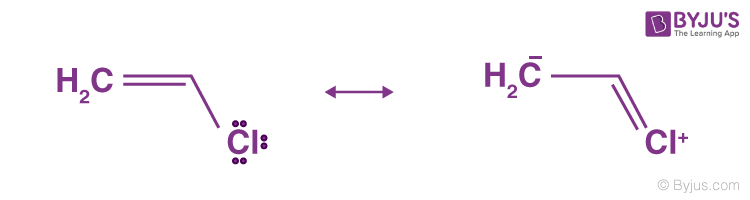The difference in the energies of the canonical forms and resonance hybrid is called resonance stabilization energy.

## Resonance in Chemical Bonding## Kossel Lewis Theory### London Dispersion Forces

Another form of chemical bonding is caused by London dispersion forces. These forces are weak in magnitude.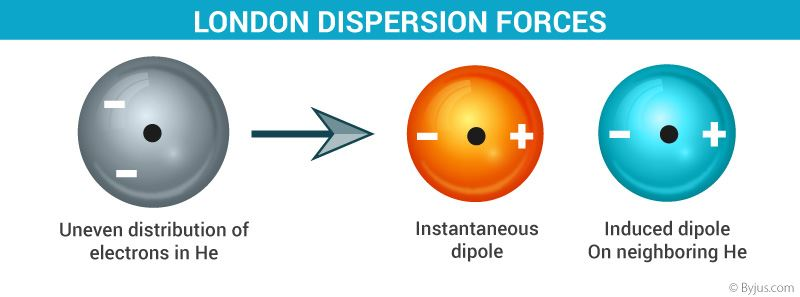Chemical Bonding – London Dispersion Forces

These forces occur due to a temporary charge imbalance arising in an atom. This imbalance in the charge of the atom can induce dipoles on neighbouring atoms. For example, the temporary positive charge on one area of an atom can attract the neighbouring negative charge.

## FAQs on Chemical Bonding and Molecular Structure

### Why atoms react and how?

Atoms having eight electrons in their last orbit are stable and have no tendency to react. Atoms having less than eight electrons, then react with other atoms to get eight electrons in their outermost orbit, and become stable. Atoms having slightly excess than eight electrons may lose them, to atoms, which, are short of eight. Atoms that cannot either loss or gain, may share to get octet configuration. Molecules short of octet configuration even after the reaction, may accept lone pair of electrons present in other atoms or molecules.

### Name the forces that keep reacting atoms together.

In metals, outer orbitals of atoms overlap and so the electrons present in them do not belong to any particular atom but flow over to all atoms as well and bind them all together (metallic bonding). Atoms that have to lose and gain electrons, becomes ions and are held together by the electrostatic forces of attraction (Ionic Bond). When atoms equally give and share electrons, the shared electrons becomes the unifying force between them (covalent bond). Electron-deficient and free lone pair containing molecules may again and satisfy the octet thirst of the electron-deficient atom. The shared electron bridges the electron-rich atom with electron-deficient atom (coordinate bond).

### What are hybridized orbitals? What are the uses of it?

Relatively similar energy sub-orbitals may merge and form a new set of the same number of orbitals, having the property of all the contributing orbitals in proportion to their numbers. These orbitals are hybridized orbitals. They are useful in explaining the similarity in bond length, bond angles, structure, shape and magnetic properties of molecules.

### sp3 and dsp2 are four hybridized orbitals. But one is the tetrahedral shape and other square planar. Why?

sp3 orbitals are formed from the s -subshell with uniform electron distribution around the nucleus and of p-subshell with distribution in the three vertical axis. Hybridized orbitals, hence have their electron distribution in three dimensions, as tetrahedral directions.

In dsp2 all the orbitals involved I hybridization have their electron distribution around the same plane. Hence, the hybridized orbitals also are in the same plane giving rise to square planar geometry.

### The oxygen molecule is paramagnetic. Is there an explanation?

Oxygen atom shares two electrons, each with another oxygen atom to form the oxygen molecule. Oxygen molecule exhibits paramagnetic nature indicating unpaired electrons. A molecular orbital theory has been proposed to explain this. According to this theory, atoms lose their orbitals and rather form an equal number of orbital covering the entire molecule and hence the name molecular orbital. Filling up of these orbitals in increasing energy order leaves unpaired electron explaining the paramagnetic behaviour of oxygen molecule.

Test your knowledge on Chemical Bonding